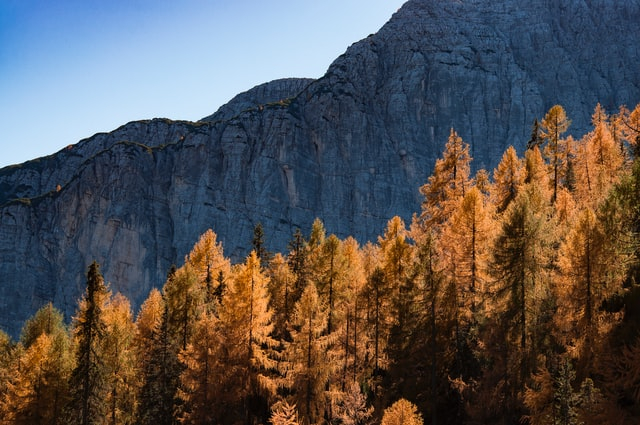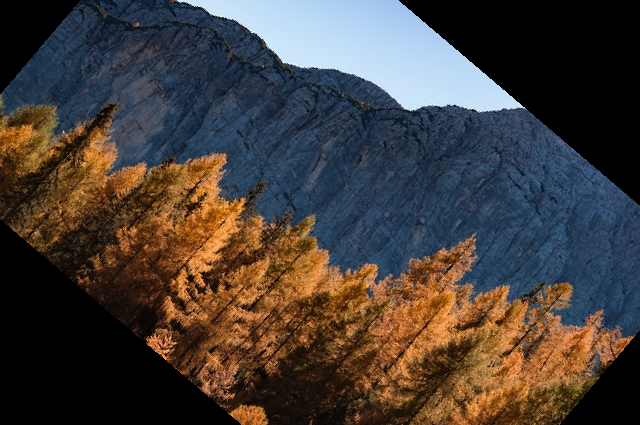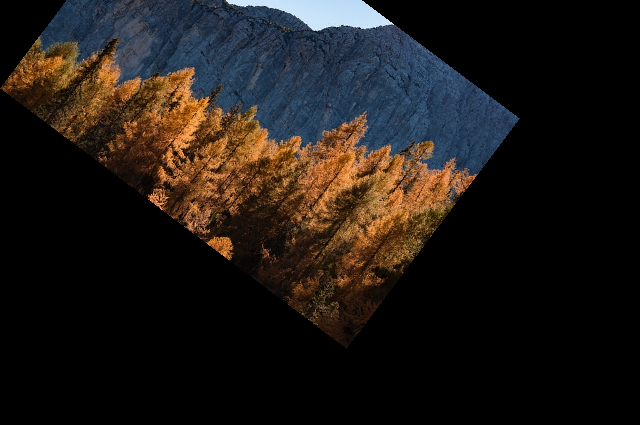# PyTorch – How to perform random affine transformation of an image?

To perform affine transformation of an image, we apply RandomAffine() transform. It's one of the many important transforms provided by the torchvision.transforms module.

RandomAffine() transformation accepts both PIL and tensor images. A tensor image is a PyTorch tensor with shape [C, H, W], where C is the number of channels, H is the image height and W is the image width.

If the image is neither a PIL image nor a tensor image, then we first convert it to a tensor image and then apply the transform.

## Syntax

torchvision.transforms.RandomAffine(degrees)(img)

## Parameters

• degrees – Desired range of degree. It's a sequence like (min, max). The image affine transformed by a degree from this range.

It returns the affine transformed image.

## Steps

We could use the following steps to perform random affine transform of an image −

• Import the required libraries. In all the following examples, the required Python libraries are torch, Pillow, and torchvision. Make sure you have already installed them.

import torch
import torchvision
import torchvision.transforms as transforms
from PIL import Image
• Read the input image. The input image is a PIL image or a Torch tensor.

img = Image.open('forest.jpg')
• Define a transform to perform random affine transform on the input image. Give the desired range of degrees.

transform = transforms.RandomAffine((30,70))
• Apply the above-defined transform on the input image to perform random affine transform.

img = transform(img)
• Visualize the affine transformed image

img.show()

Note − In the following examples, you may get an output image transformed with different degrees because RandomAffine() transform randomly chooses a degree from a given range of degrees. For example, for degrees = (30, 70), the degree can be any value in the range (30, 70).

## Input Image

The following image is used as the input in all the examples.## Example 1

The following Python3 program shows how to perform random affine transform on a PIL image.

# import requierd libraries
import torch
from PIL import Image
import torchvision.transforms as transforms

img = Image.open('forest.jpg')

# define the transform to apply random affine
transform = transforms.RandomAffine((30, 70))

# apply the above defined transform on the input image
img = transform(img)

# display the transformed image
img.show()

## Output

It will produce the following output image −## Example 2

In the following Python3 program, we translate and scale the image along with affine transformation.

# import required libraries
import torch
from PIL import Image
import torchvision.transforms as transforms

img = Image.open('forest.jpg')

# define the transform to apply random affine
transform = transforms.RandomAffine(degrees=(30, 70),
translate=(0.1, 0.3), scale=(0.5, 0.75))

# Apply the above defined transform
img = transform(img)

# display the transformed image
img.show()

## Output

It will produce the following output −